Loading 2022 NFL Offensive Rookie of the Year Futures...
2022 NFL Offensive Rookie of the Year Odds
+500
5 to 1Chris Olave
10.8% implied probability

+500
5 to 1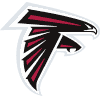Drake London
10.8% implied probability

+800
8 to 1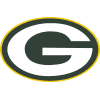Romeo Doubs
7.2% implied probability

+1000
10 to 1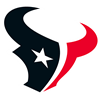Dameon Pierce
5.9% implied probability

+1200
12 to 1Garrett Wilson
5.0% implied probability

+1400
14 to 1Jahan Dotson
4.3% implied probability

+1500
15 to 1Kenny Pickett
4.0% implied probability

+1500
15 to 1Breece Hall
4.0% implied probability

+1600
16 to 1George Pickens
3.8% implied probability

+1800
18 to 1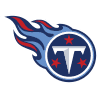Treylon Burks
3.4% implied probability

+2000
20 to 1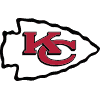Isiah Pacheco
3.1% implied probability

+2200
22 to 1Brian Robinson
2.8% implied probability

+3000
30 to 1Malik Willis
2.1% implied probability

+3500
35 to 1James Cook
1.8% implied probability

+4000
40 to 1Desmond Ridder
1.6% implied probability

+4000
40 to 1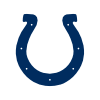Jelani Woods
1.6% implied probability

+5000
50 to 1Alec Pierce
1.3% implied probability

+5000
50 to 1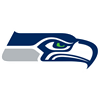Kenneth Walker
1.3% implied probability

+5000
50 to 1Kyle Phillips
1.3% implied probability

+5000
50 to 1Rachaad White
1.3% implied probability

+5000
50 to 1Christian Watson
1.3% implied probability

+5500
55 to 1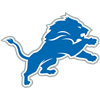Jameson Williams
1.2% implied probability

+6500
65 to 1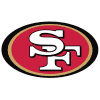Tyrion Davis-Price
1.0% implied probability

+7000
70 to 1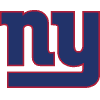Wan'Dale Robinson
0.9% implied probability

+7000
70 to 1Tyler Allgeier
0.9% implied probability

+7500
75 to 1Isaiah Likely
0.8% implied probability

+8000
80 to 1Dennis Houston
0.8% implied probability

+8000
80 to 1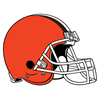David Bell
0.8% implied probability

+10000
100 to 1Jordan Mason
0.6% implied probability

+10000
100 to 1KaVontae Turpin
0.6% implied probability

+10000
100 to 1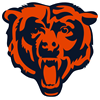Velus Jones
0.6% implied probability

+10000
100 to 1Kyren Williams
0.6% implied probability

+10000
100 to 1Dontario Drummond
0.6% implied probability

+10000
100 to 1Jalen Tolbert
0.6% implied probability

+10000
100 to 1Skyy Moore
0.6% implied probability

+10000
100 to 1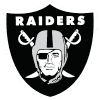Zamir White
0.6% implied probability

+10000
100 to 1Sam Howell
0.6% implied probability

+10000
100 to 1Trey McBride
0.6% implied probability

+10000
100 to 1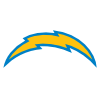Isaiah Spiller
0.6% implied probability

+12000
120 to 1Calvin Austin
0.5% implied probability

+12000
120 to 1Khalil Shakir
0.5% implied probability

+12000
120 to 1Tyquan Thornton
0.5% implied probability

+13000
130 to 1Zonovan Knight
0.5% implied probability

+15000
150 to 1Tyler Goodson
0.4% implied probability

+15000
150 to 1Jerome Ford
0.4% implied probability

+15000
150 to 1Cade Otton
0.4% implied probability

+15000
150 to 1Kaleb Eleby
0.4% implied probability

+15000
150 to 1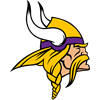Ty Chandler
0.4% implied probability

+15000
150 to 1Hassan Haskins
0.4% implied probability

+20000
200 to 1ZaQuandre White
0.3% implied probability

+20000
200 to 1Ikem Ekwonu
0.3% implied probability

+20000
200 to 1Bailey Zappe
0.3% implied probability

+20000
200 to 1Pierre Strong
0.3% implied probability

+20000
200 to 1Jerrion Ealy
0.3% implied probability

+20000
200 to 1Cole Turner
0.3% implied probability

+20000
200 to 1Charles Cross
0.3% implied probability

+20000
200 to 1Evan Neal
0.3% implied probability

+20000
200 to 1Lance McCutcheon
0.3% implied probability

+20000
200 to 1Tyler Linderbaum
0.3% implied probability

+20000
200 to 1Jeremy Ruckert
0.3% implied probability